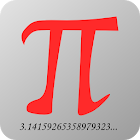Math - mathematics is easy

All Android applications categories

All Android games categories# Math - mathematics is easy

by: 3.7K 8.4

8.4 Users
rating

## Screenshots

Description

Math on the phone!

Now you have all useful math formulas and definitions handy and can always refer to them. There are a lot of drawings (including 3D), explaining all symbols used in formulas.
Range of material - high school.

The content is divided into sections and subsections so that it is easy to find any formula, definition or equation.

Many people have asked about integrals - already are, but here only at the secondary level. Advanced integrals are already available in a twin Matma2 application dedicated to the students.

There is also a paid version of the Math (MathPro), which does not show ads, and includes material from both Math and Math2 apps.

Algebra
- Constants
- Basics
- Exponents
- Averages
- Polynomials
- Logarithms
- Equations
- Equation systems
- Matrices
- Determinants
Analysis
- Sequences
- Limits
- Series
- Integrals properties
- Integrals, basic formulas
Geometry
- Triangles
- Trigonometry
- Polygons
- Circles
- Polyhedrons
- Solids of revolution
- Platonic Solids
Statistics
- Combinatorics
- Probability
Logic, Sets
- Logic

Coming soon: vector calculus, differential calculus, analytic geometry, complex numbers, ... then the next sections.

Tags: ma/matma pl , math application , math , differential math , matma wzory skroconego mnozenia , lication math , math formula lications , całki , math applidation

## Users review

from 3.723 reviews

"Great"

8.4